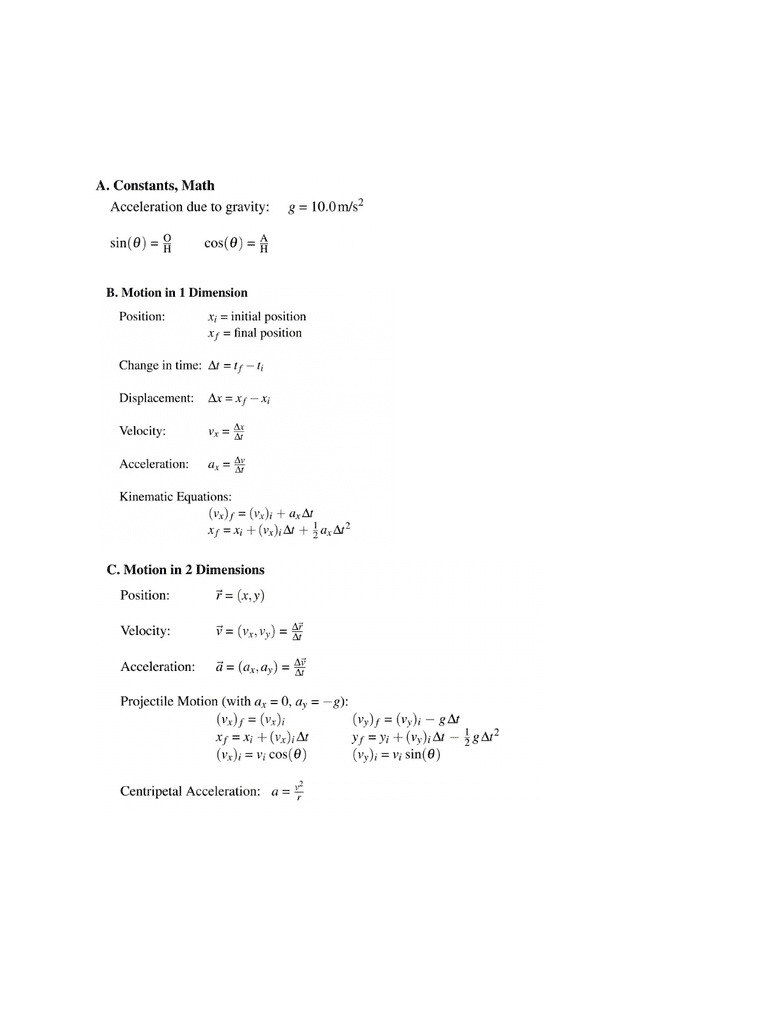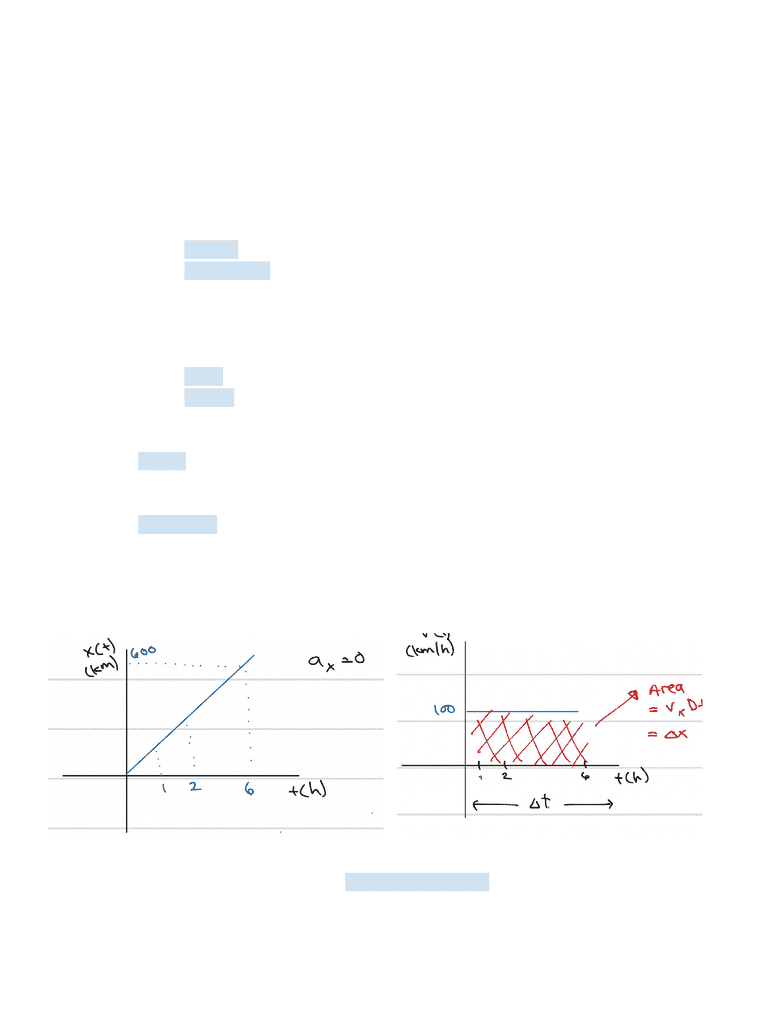Get 2 days of premium access
Study Guides (380,000)
US (220,000)
UCLA (3,000)
PHYSICS (70)
Midterm

# PHYSICS 5A Study Guide - Midterm Guide: Minor Places In Arda

Department
Physics
Course Code
PHYSICS 5A
Professor
Josh Samani
Study Guide
Midterm

This preview shows pages 1-2. to view the full 6 pages of the document.Physics 5a_ Study Guide_ Midterm 1
Formulas that professor provided ( images are from CCLE, professor recommends studying
the formula sheet and familiarize yourself with the syntax and equations.
KNOW what each constant represents. When working out problem, see with variables you
have and then find the equations that allow you to plug in the most number while also
allowing you to find what you’re looking for. Note that sometimes you have to use more than
one formulas to get to your answer

Only pages 1-2 are available for preview. Some parts have been intentionally blurred.Concepts for Motion
Action that is happen in the x direction has no effect of the y direction
Object moving to the right has no effect on the object being thrown upward
Remember to use signs (+) and (-) to indicate the direction that the object is going in.
For gravity, object falling down is (-g), object being thrown up is (+g)
Difference between position and displacement
Position = total distance traveled
Displacement= final position - initial position
Ex: From point A to point B is 50 m. If Ned walked from point A to B and
then B to A, his total position is 100 m. His displacement is 0 because
50-50 =0
Difference between speed and velocity
Speed = describe how fast an object is moving
Velocity = describe how fast an object is going and the direction it is going in
When an object is being thrown up into the air, it’s velocity will always be
decreasing
Vectors: has magnitude and direction and is represented by an arrow.
In circular motion, direction can change, but velocity stays the same (constant).
Meaning speed can change, when velocity is constant.
Acceleration: the rate of change in velocity over the change in time.
Motion in 2D
Velocity vs. Time graph and Position vs. Time Graph
Velocity vs. Time graph shows you the slope of the graph while Position vs. Time
graph shows you the displacement of the object (area under the curve)
Difference between velocity and instantaneous velocity : the instantaneous speed is
actually the real definition of velocity. The difference is that normal velocity is assumed
###### You're Reading a Preview

Unlock to view full version